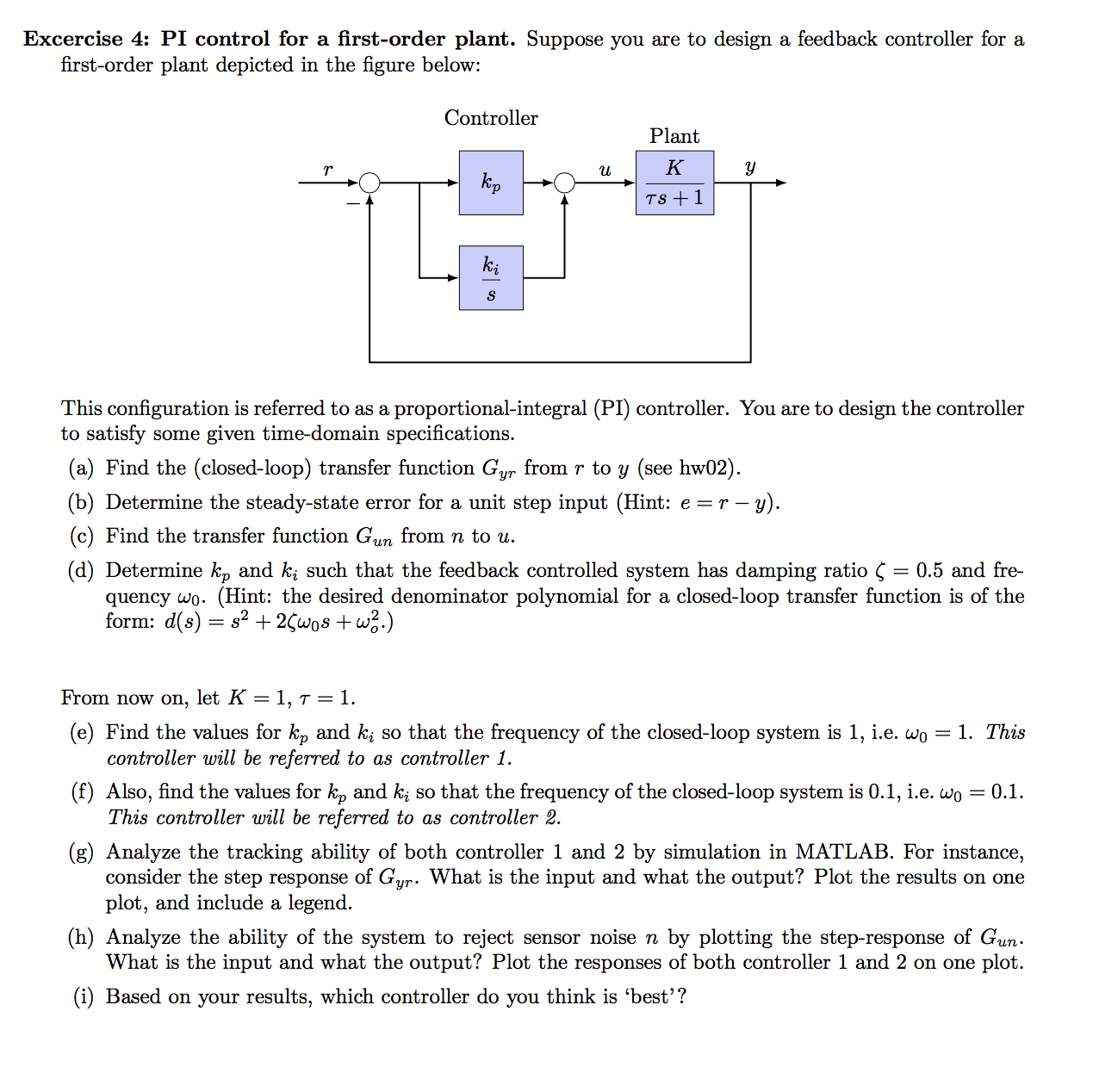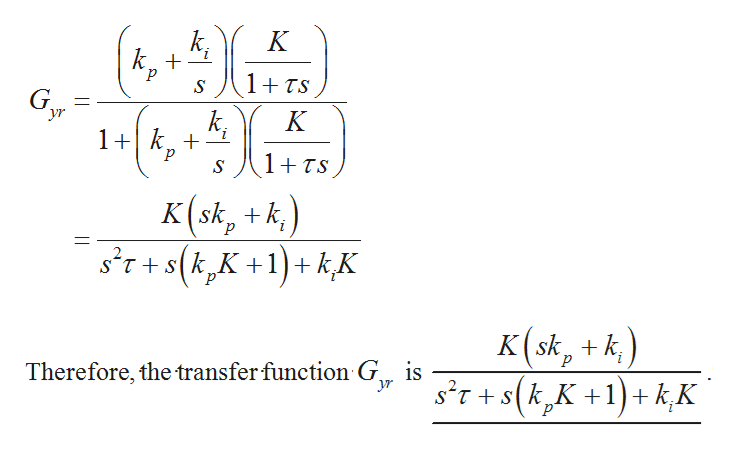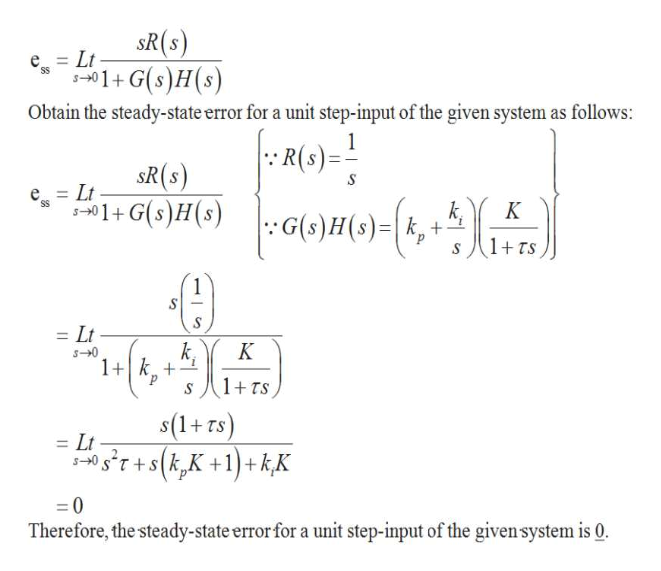# Excercise 4: PI control for a first-order plant. Suppose you are to design a feedback controller for afirst-order plant depicted in the figure below:ControllerPlantКkpTS 1SThis configuration is referred to as a proportional-integral (PI) controller. You are to design the controllerto satisfy some given time-domain specifications.(a) Find the (closed-loop) transfer function Gyr from r to y (see hw02).(b) Determine the steady-state error for a unit step input (Hint: e r --y)(c) Find the transfer function Gun from n to u.(d) Determine k, and ki such that the feedback controlled system has damping ratio Ç = 0.5 and fre-quency wo. (Hint: the desired denominator polynomial for a closed-loop transfer function is of theform: d(s) s2+ 2Çwos +w2.)From now on, let K = 1, t = 1.(e) Find the values for kp and ki so that the frequency of the closed-loop system is 1, i.e. wo = 1. Thiscontroller will be referred to as controller 1.(f) Also, find the values for k, and k so that the frequency of the closed-loop system is 0.1, i.e. woThis controller will be referred to as controller 2.= 0.1(g) Analyze the tracking ability of both controller 1 and 2 by simulation in MATLAB. For instance,consider the step response of Gur. What is the input and what the output? Plot the results on oneplot, and include a legend(h) Analyze the ability of the system to reject sensor noise n by plotting the step-response of GunWhat is the input and what the output? Plot the responses of both controller 1 and 2 on one plot.(i) Based on your results, which controller do you think is 'best'?

Question
4 viewshelp_outlineImage TranscriptioncloseExcercise 4: PI control for a first-order plant. Suppose you are to design a feedback controller for a first-order plant depicted in the figure below: Controller Plant К kp TS 1 S This configuration is referred to as a proportional-integral (PI) controller. You are to design the controller to satisfy some given time-domain specifications. (a) Find the (closed-loop) transfer function Gyr from r to y (see hw02). (b) Determine the steady-state error for a unit step input (Hint: e r - -y) (c) Find the transfer function Gun from n to u. (d) Determine k, and ki such that the feedback controlled system has damping ratio Ç = 0.5 and fre- quency wo. (Hint: the desired denominator polynomial for a closed-loop transfer function is of the form: d(s) s2+ 2Çwos +w2.) From now on, let K = 1, t = 1. (e) Find the values for kp and ki so that the frequency of the closed-loop system is 1, i.e. wo = 1. This controller will be referred to as controller 1. (f) Also, find the values for k, and k so that the frequency of the closed-loop system is 0.1, i.e. wo This controller will be referred to as controller 2. = 0.1 (g) Analyze the tracking ability of both controller 1 and 2 by simulation in MATLAB. For instance, consider the step response of Gur. What is the input and what the output? Plot the results on one plot, and include a legend (h) Analyze the ability of the system to reject sensor noise n by plotting the step-response of Gun What is the input and what the output? Plot the responses of both controller 1 and 2 on one plot. (i) Based on your results, which controller do you think is 'best'? fullscreen
check_circle

Step 1

Hi! Thank you for the question. I am solving the Question for first 3 sub-parts as you have posted the question with multiple sub-parts. Kindly post the remaining sub-parts as separate Question. Thank you.

Part (a):

The closed-loop transfer function from r to y is obtained as follows:

Step 2

From Figure 2, obtain the required closed-loop transfer function from r to y as follows:help_outlineImage TranscriptioncloseК k + p 1+TS 1(,-5)6 yr К 1+k 1+TS S K(sk, +k) s'T+s(kK+1)+kK p K(sk, k sr+s(kK1)k,K Therefore, the transfer function G is K+. fullscreen
Step 3

Part (b):

Write the expression for steady-state erro...help_outlineImage TranscriptioncloseSR(s) 1G(s)H(s) =Lt SS Obtain the steady-state error for a unit step-input of the given system as follows: tR(s) = SR(8) S Lt е 3 aoma-(e,)X5) 1+G(s)H(s) k G(s)H(s)k + 1+ TS =Lt k К s-0 1+k+ 1+TS s(1+ rs) K+1) +kK = Lt s-0 sT+sk 0 Therefore, the steady-state error for a unit step-input of the given system is 0. fullscreen

### Want to see the full answer?

See Solution

#### Want to see this answer and more?

Solutions are written by subject experts who are available 24/7. Questions are typically answered within 1 hour.*

See Solution
*Response times may vary by subject and question.
Tagged in

### Electrical Engineering